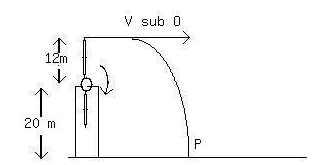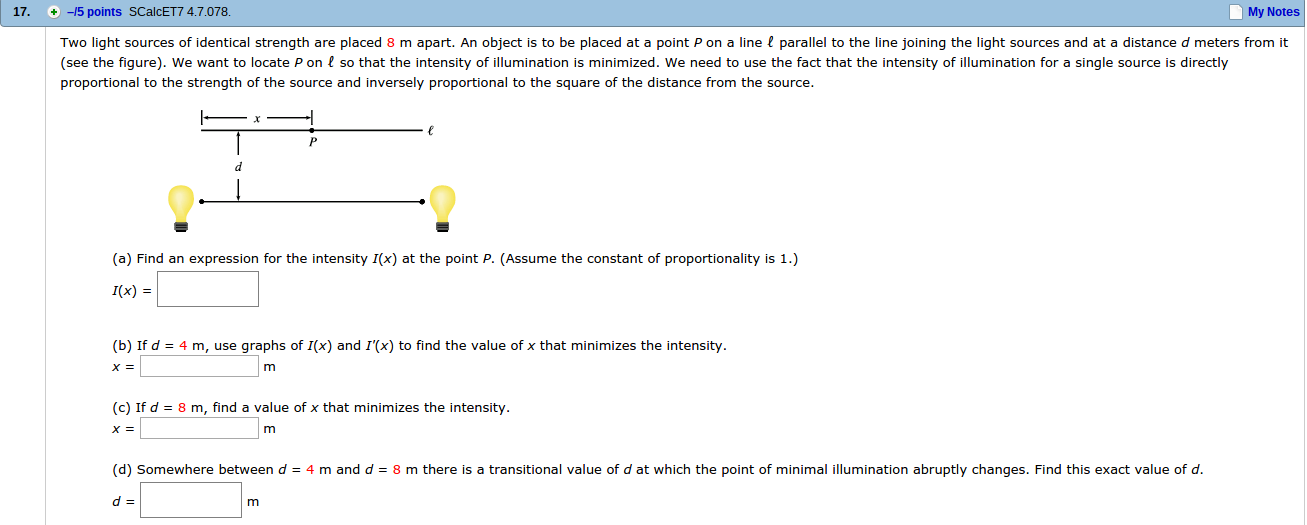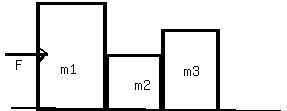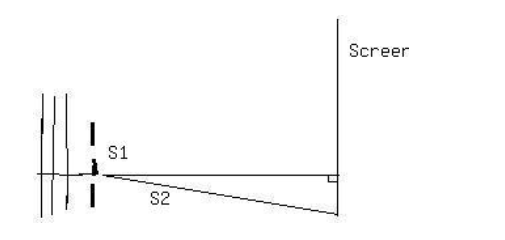Ask question

# Draw a diagram to represent the situation. Start by drawing a segment to represent the distance between Sam and the base of the building. Place a point on the segment and label the portion from Sam to this point as 1.22 m and the other portion as 7.32 m. Then draw two right triangles that have right angles at the endpoints of the first segment. Label the height for Sam's triangle as 1.82 m and the height of the building's triangle as h:# Draw a diagram to represent the situation. Start by drawing a segment to represent the distance between Sam and the base of the building. Place a point on the segment and label the portion from Sam to this point as 1.22 m and the other portion as 7.32 m. Then draw two right triangles that have right angles at the endpoints of the first segment. Label the height for Sam's triangle as 1.82 m and the height of the building's triangle as h:

Question
Fractionsasked 2021-01-27
Draw a diagram to represent the situation. Start by drawing a segment to represent the distance between Sam and the base of the building. Place a point on the segment and label the portion from Sam to this point as 1.22 m and the other portion as 7.32 m. Then draw two right triangles that have right angles at the endpoints of the first segment. Label the height for Sam's triangle as 1.82 m and the height of the building's triangle as h:

## Answers (1)2021-01-28
The angle of reflection is the same from the mirror to Sam and from the mirror to the window so the two triangles are similar. The corresponding side lengths must then be proportional. Therefore:
$$\displaystyle{\frac{{{1.82}}}{{{1.22}}}}={\frac{{{h}}}{{{7.32}}}}$$
$$\displaystyle{\frac{{{1.82}}}{{{7.32}}}}={1.22}{h}$$
$$\displaystyle{13.3224}={1.22}{h}$$
$$\displaystyle{10.92}=\frac{{h}}{{7}}$$
[Pic]
The height of the window is then 10.92 m.

### Relevant Questionsasked 2021-03-26
A helicopter carrying dr. evil takes off with a constant upward acceleration of $$\displaystyle{5.0}\ \frac{{m}}{{s}^{{2}}}$$. Secret agent austin powers jumps on just as the helicopter lifts off the ground. Afterthe two men struggle for 10.0 s, powers shuts off the engineand steps out of the helicopter. Assume that the helicopter is infree fall after its engine is shut off and ignore effects of airresistance.
a) What is the max height above ground reached by the helicopter?
b) Powers deploys a jet pack strapped on his back 7.0s after leaving the helicopter, and then he has a constant downward acceleration with magnitude 2.0 m/s2. how far is powers above the ground when the helicopter crashes into the ground.asked 2021-04-25
The unstable nucleus uranium-236 can be regarded as auniformly charged sphere of charge Q=+92e and radius $$\displaystyle{R}={7.4}\times{10}^{{-{15}}}$$ m. In nuclear fission, this can divide into twosmaller nuclei, each of 1/2 the charge and 1/2 the voume of theoriginal uranium-236 nucleus. This is one of the reactionsthat occurred n the nuclear weapon that exploded over Hiroshima, Japan in August 1945.
A. Find the radii of the two "daughter" nuclei of charge+46e.
B. In a simple model for the fission process, immediatelyafter the uranium-236 nucleus has undergone fission the "daughter"nuclei are at rest and just touching. Calculate the kineticenergy that each of the "daughter" nuclei will have when they arevery far apart.
C. In this model the sum of the kinetic energies of the two"daughter" nuclei is the energy released by the fission of oneuranium-236 nucleus. Calculate the energy released by thefission of 10.0 kg of uranium-236. The atomic mass ofuranium-236 is 236 u, where 1 u = 1 atomic mass unit $$\displaystyle={1.66}\times{10}^{{-{27}}}$$ kg. Express your answer both in joules and in kilotonsof TNT (1 kiloton of TNT releases 4.18 x 10^12 J when itexplodes).asked 2021-04-24A wind farm generator uses a two-bladed propellermounted on a pylon at a height of 20 m. The length of eachpropeller blade is 12 m. A tip of the propeller breaks offwhen the propeller is vertical. The fragment flies offhorizontally, falls, and strikes the ground at P. Just beforethe fragment broke off, the propeller was turning uniformly, taking1.2 s for each rotation. In the above figure, the distancefrom the base of the pylon to the point where the fragment strikesthe ground is closest to:
a) 130 m
b) 160 m
c) 120 m
d) 140 m
e) 150 masked 2021-02-19
A 10 kg objectexperiences a horizontal force which causes it to accelerate at 5 $$\displaystyle\frac{{m}}{{s}^{{2}}}$$, moving it a distance of 20 m, horizontally.How much work is done by the force?
A ball is connected to a rope and swung around in uniform circular motion.The tension in the rope is measured at 10 N and the radius of thecircle is 1 m. How much work is done in one revolution around the circle?
A 10 kg weight issuspended in the air by a strong cable. How much work is done, perunit time, in suspending the weight?
A 5 kg block is moved up a 30 degree incline by a force of 50 N, parallel to the incline. The coefficient of kinetic friction between the block and the incline is .25. How much work is done by the 50 N force in moving the block a distance of 10 meters? What is the total workdone on the block over the same distance?
What is the kinetic energy of a 2 kg ball that travels a distance of 50 metersin 5 seconds?
A ball is thrown vertically with a velocity of 25 m/s. How high does it go? What is its velocity when it reaches a height of 25 m?
A ball with enough speed can complete a vertical loop. With what speed must the ballenter the loop to complete a 2 m loop? (Keep in mind that the velocity of the ball is not constant throughout the loop).asked 2021-04-21
The crane shown in the drawing is lifting a 182-kg crate upward with an acceleration of $$\displaystyle{1.5}\frac{{m}}{{s}^{{2}}}$$. The cable from the crate passes over a solid cylindrical pulley at the top of the boom. The pulley has a mass of 130 kg. The cable is then wound ontoa hollow cylindrical drum that is mounted on the deck of the crane.The mass of the drum is 150 kg, and its radius is 0.76 m. The engine applies a counter clockwise torque to the drum in order towind up the cable. What is the magnitude of this torque? Ignore the mass of the cable.asked 2021-02-10
Two light sources of identical strength are placed 8 m apart. An object is to be placed at a point P on a line ? parallel to the line joining the light sources and at a distance d meters from it (see the figure). We want to locate P on ? so that the intensity of illumination is minimized. We need to use the fact that the intensity of illumination for a single source is directly proportional to the strength of the source and inversely proportional to the square of the distance from the source.asked 2021-03-24
The figure shows 3 crates being pushed over a concrete floor by a horizontal force f of magnitude 440N. The masses of the cratesare $$\displaystyle{m}_{{1}}={30}$$ kg, $$\displaystyle{m}_{{2}}={10}$$ kg, and $$\displaystyle{m}_{{3}}={20}$$ kg.The coefficient of kineticfriction between the floor and each of the crates is 0.7. a) what is the magnitude $$\displaystyle{F}_{{{32}}}$$ of the force on crate 3 from crate 2? b) If the crates then slide onto a polished floor, where the coefficientof kinetic friction is less than 0.700, is magnitude PSKF_{32}ZSL more than,less than, or the same as it was when the coeffient was 0.700?asked 2021-05-181. S1 and S2, shown above, are thin parallel slits in an opaqueplate. A plane wave of wavelength λ is incident from the leftmoving in a direction perpendicular to the plate. On a screenfar from the slits there are maximums and minimums in intensity atvarious angles measured from the center line. As the angle isincreased from zero, the first minimum occurs at 3 degrees. Thenext minimum occurs at an angle of-
A. 4.5 degrees
B. 6 degrees
C. 7.5 degrees
D. 9 degrees
E. 12 degreesasked 2021-03-22
A box is sliding with a speed of 4.50 m/s on a horizontal surface when, at point P, it encounters a rough section. On the rough section, the coefficient of friction is not constant but starts at .100 at P and increases linerly with distance past P, reaching a value of .600 at 12.5 m past point P. (a) Use the work energy theorem to find how far this box slides before stopping. (b) What is the coefficient of friction at the stopping point? (c) How far would the box have slid iff the friciton coefficient didn't increase, but instead had the constant value of .1?asked 2021-03-07
This problem is about the equation
dP/dt = kP-H , P(0) = Po,
where k > 0 and H > 0 are constants.
If H = 0, you have dP/dt = kP , which models expontialgrowth. Think of H as a harvesting term, tending to reducethe rate of growth; then there ought to be a value of H big enoughto prevent exponential growth.
Problem: find acondition on H, involving $$\displaystyle{P}_{{0}}$$ and k, that will prevent solutions from growing exponentially.
...